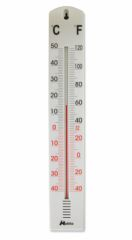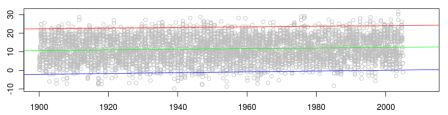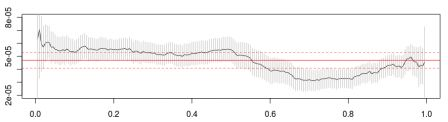# Warming in Paris: minimas versus maximas ?

January 14, 2011
By

Want to share your content on R-bloggers? click here if you have a blog, or here if you don't.Recently, I received comments (here and on Twitter) about my previous graphs on the
temperature in Paris. I mentioned in a comment (there) that studying
extremas (and more generally quantiles
or interquantile evolution) is not the same as studying the variance.
Since I am not a big fan of the variance, let us talk a little bit
In order to study the average temperature it is natural to look at the
linear (assuming that it is linear, but I proved that it could
reasonably be assumed as linear in the paper) regression, i.e. least
square regression, which gives the expected value. But if we care about
extremes, or almost extremes, it is natural to look at quantile
regression.
For instance, below, the green line
is the least square regression, the red one is 97.5%
quantile,
and the blue
on the 2.5% quantile regression.It looks like the slope is the same, i.e. extremas are increasing as
fast as the average…

`tmaxparis=read.table("temperature/TG_SOUID100845.txt",skip=20,sep=",",header=TRUE)head(tmaxparis)Dparis=as.Date(as.character(tmaxparis\$DATE),"%Y%m%d")Tparis=as.numeric(tmaxparis\$TG)/10Tparis[Tparis==-999.9]=NAI=sample(1:length(Tparis),size=5000,replace=FALSE)plot(Dparis[I],Tparis[I],col="grey")abline(lm(Tparis~Dparis),col="green")library(quantreg)abline(rq(Tparis~Dparis,tau=.025),col="blue")abline(rq(Tparis~Dparis,tau=.975),col="red")`

(here I plot randomly some points to avoid a too heavy figure, since I
have too many observations, but I keep all the observations in the
regression !).
Now, if we look at the slope for different quantile level (Fig 6 in the
paper, here,
but on minimum daily temperature, here I look at average daily
temperature), the interpretation is different.

`s=0COEF=SD=rep(NA,199)for(i in seq(.005,.995,by=.005)){s=s+1REG=rq(Tparis~Dparis,tau=i)COEF[s]=REG\$coefficientsSD[s]=summary(REG)\$coefficients[2,2]}`

with the following graph below,

`s=0plot(seq(.005,.995,by=.005),COEF,type="l",ylim=c(0.00002,.00008))for(i in seq(.005,.995,by=.005)){s=s+1segments(i,COEF[s]-2*SD[s],i,COEF[s]+2*SD[s],col="grey")}REG=lm(Tparis~Dparis)COEFlm=REG\$coefficientsSDlm=summary(REG)\$coefficients[2,2]abline(h=COEFlm,col="red")abline(h=COEFlm-2*SDlm,lty=2,lw=.6,col="red")abline(h=COEFlm+2*SDlm,lty=2,lw=.6,col="red")`Here, for minimas (quantiles associated to low probabilities, on the left), the
trend has a higher slope than the average, so in some sense, warming of
minimas is stronger than average temperature, and on other hand, for
maximas (high probabilities on
the right), the slope is smaller – but positive – so summer are warmer,
but not as much as winters.
Note also that the story is different for minimal temperature
(mentioned in the paper) compared with that study, made here on average
daily temperature (see comments)… This is not a major breakthrough in climate research, but this is all I got…

R-bloggers.com offers daily e-mail updates about R news and tutorials about learning R and many other topics. Click here if you're looking to post or find an R/data-science job.
Want to share your content on R-bloggers? click here if you have a blog, or here if you don't.

Tags: , , , , , , ,